Chapter 8.3, Problem 3EEMathematical Excursions (MindTap C...

4th Edition
Richard N. Aufmann + 3 others
ISBN: 9781305965584

Solutions

Chapter
SectionMathematical Excursions (MindTap C...

4th Edition
Richard N. Aufmann + 3 others
ISBN: 9781305965584
Textbook Problem

In Figure 8.6, determine where the black cat will be after applying the following translations illustrated in Figure 8.4.a. A Δ ( D Δ C ) b. ( C Δ D ) Δ A c. ( A Δ H ) Δ ( E Δ D )a.

To determine

In figure 1, where the black cat will be after applying the translations FΔ(DΔC) illustrated in figure 2.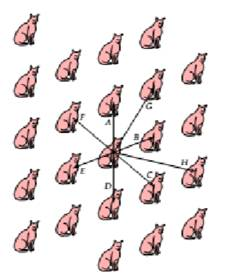Figure 1  Figure 2

Explanation

Given information: The translation is FΔ(DΔC)

Solution:

A

b.

To determine

In figure 1, where the black cat will be after applying the translations (CΔD)ΔG illustrated in figure 2.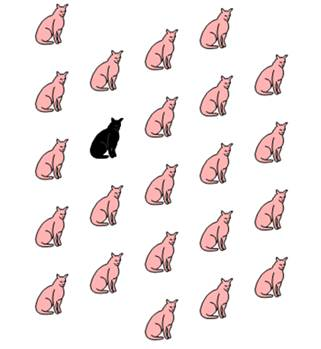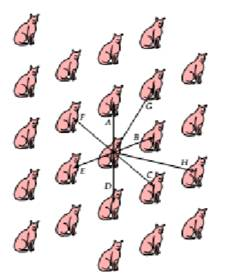Figure 1   Figure 2

c.

To determine

In figure 1, where the black cat will be after applying the translations (AΔH)Δ(EΔD) illustrated in figure 2.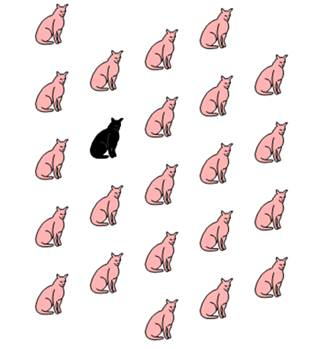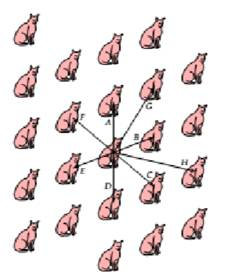Figure 1  Figure 2

Still sussing out bartleby?

Check out a sample textbook solution.

See a sample solution

The Solution to Your Study Problems

Bartleby provides explanations to thousands of textbook problems written by our experts, many with advanced degrees!

Get Started

Solve 123x3x+22 and graph the solution.

Mathematical Applications for the Management, Life, and Social Sciences

Evaluate the expression sin Exercises 116. (42)2

Finite Mathematics and Applied Calculus (MindTap Course List)

Describe the scores in a sample that has standard deviation of zero.

Statistics for The Behavioral Sciences (MindTap Course List)

Determine the infinite limit. limx0+ln(sinx)

Single Variable Calculus: Early Transcendentals, Volume I